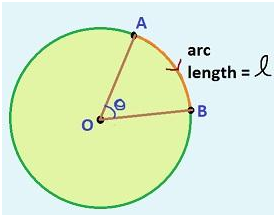# Angle Formula

An angle is formed when two rays intersect, i.e. half-lines projected with a common endpoint. The corner point of angle is known as the vertex of the angle and the rays as the sides, i.e. the lines are known as the arms. It is defined as the measure of turn between the two lines. The unit of angle is radians or degrees. There are different types of formulas for angles some of them are double-angle formula, half-angle formula, compound angle formula, interior angle formula etc.Central Angle Formula

$\large Angle = \frac{Arc\: Length \times 360^o}{2\pi Radius}$

Formula for Central Angle

$\large s = r\theta$

θ = s/r
Here, θ
represents the central angle in radians, s represents the arc length and r is the length of the radius.

Formula for Double Angle

$\large \cos (2a) = \cos ^{2}(a) – \sin ^{2}(a) = 2 \cos ^{2}(a) -1 = 1-2\sin ^{2}(a)$ $\large \sin (2a) = 2\sin (a)\cos (a)$ $\large \tan (2a) = \frac{2\tan(a)}{1-\tan ^{2}(a)}$

### Solved Example

Example : Find the angle of a segment in a circle if the arc length is 5π and the radius is 6 units.
Solution:

Using the central angle formula,
Angle =
$$\begin{array}{l}\frac{Arc Length \times 360^o}{2 \times \pi \times Radius}\end{array}$$

Angle =
$$\begin{array}{l}\frac{5 \pi \times 360}{2 \times \pi \times 6}\end{array}$$
degrees
Angle = 150 degrees
 More topics in Angle Formulas Radians to Degrees Formula Multiple Angle Formulas Right Angle Formula